# XOR Function

Returns a logical Exclusive OR of all arguments

## What is the XOR Function?

The XOR Function was introduced in Excel 2013 and is available under Excel Logical functions. It is a logical “exclusive OR” function. For two given logical statements, the XOR function would return TRUE if one of the statements is true and FALSE if both statements are true. If neither of the statements is true, it also returns FALSE.

### Formula

= XOR(logical1, [logical2],…)

The XOR function includes the following arguments:

Logical1, logical2 – Logical1 is a required argument, whereas logical2 and subsequent logical values are optional.

### How to use the XOR Function in Excel?

The XOR Function, as mentioned above, returns logical results. It will provide the following results in different scenarios: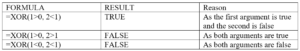In case of more than two logical statements, the function will return:

1. TRUE – When an odd number of the arguments evaluates to TRUE;
2. FALSE – When all statements are false or when the total number of TRUE statements is even.

To understand the uses of this function and how it can be used, let’s consider few examples:

#### Example 1

Using the XOR function, we would get the following results: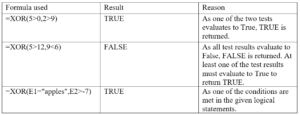A screenshot of the results in Excel is shown below: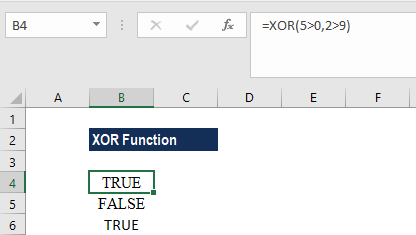#### Example 2

Suppose there are six international teams playing in a hockey tournament. Each team has already played two games. We have the results of their first two games and we now wish to find out which team will play the third game based on the following conditions of the tournament:

1. Teams who won both games qualify for the final.
2. Teams who lost both the games are out of the tournament.
3. Teams who won only one game out of the two are required to play the third game to determine which team goes into the next round and which team doesn’t.

The results of the first two games are as follows: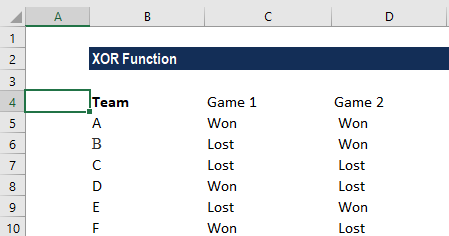Now, to find out if the team should play the third game, we can use the simple formula =XOR(C5=”Won”, D5=”Won”). However, we can also nest it using the IF formula as shown below: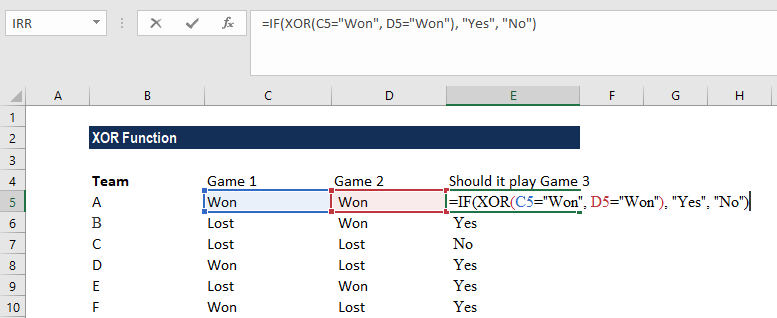We get following results: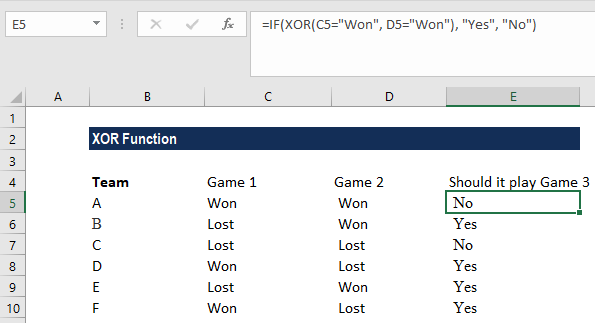The formula used here is =IF(XOR(B2=”Won”, C2=”Won”), “Yes”, “No”). Thus, XOR made it easy to pair up teams.

Generally, XOR is used along with another logical function. Adding the IF Formula will do one thing if a condition is TRUE, and will do another if a condition is FALSE. Often when using the OR Function, we shall “nest” it within an IF Function.

The other logical functions that can be used are AND functions, which test if all conditions are met. We can also use OR formula to test if one or more conditions are met.

### Things to remember about the XOR Function

1. The XOR Function allows us to test up to 254 functions.
2. XOR ignores empty references.
3. #VALUE! error will be returned when logical values are not found.
4. #NAME! error will be returned when the function is used in Excel versions earlier than 2013.
5. Arguments should be evaluated to logical values such as TRUE or FALSE, or in arrays or references that contain logical values.

Thanks for reading CFI’s guide to important Excel functions! By taking the time to learn and master these functions, you’ll significantly speed up your financial analysis. To learn more, check out these additional CFI resources:

• Excel Functions for Finance